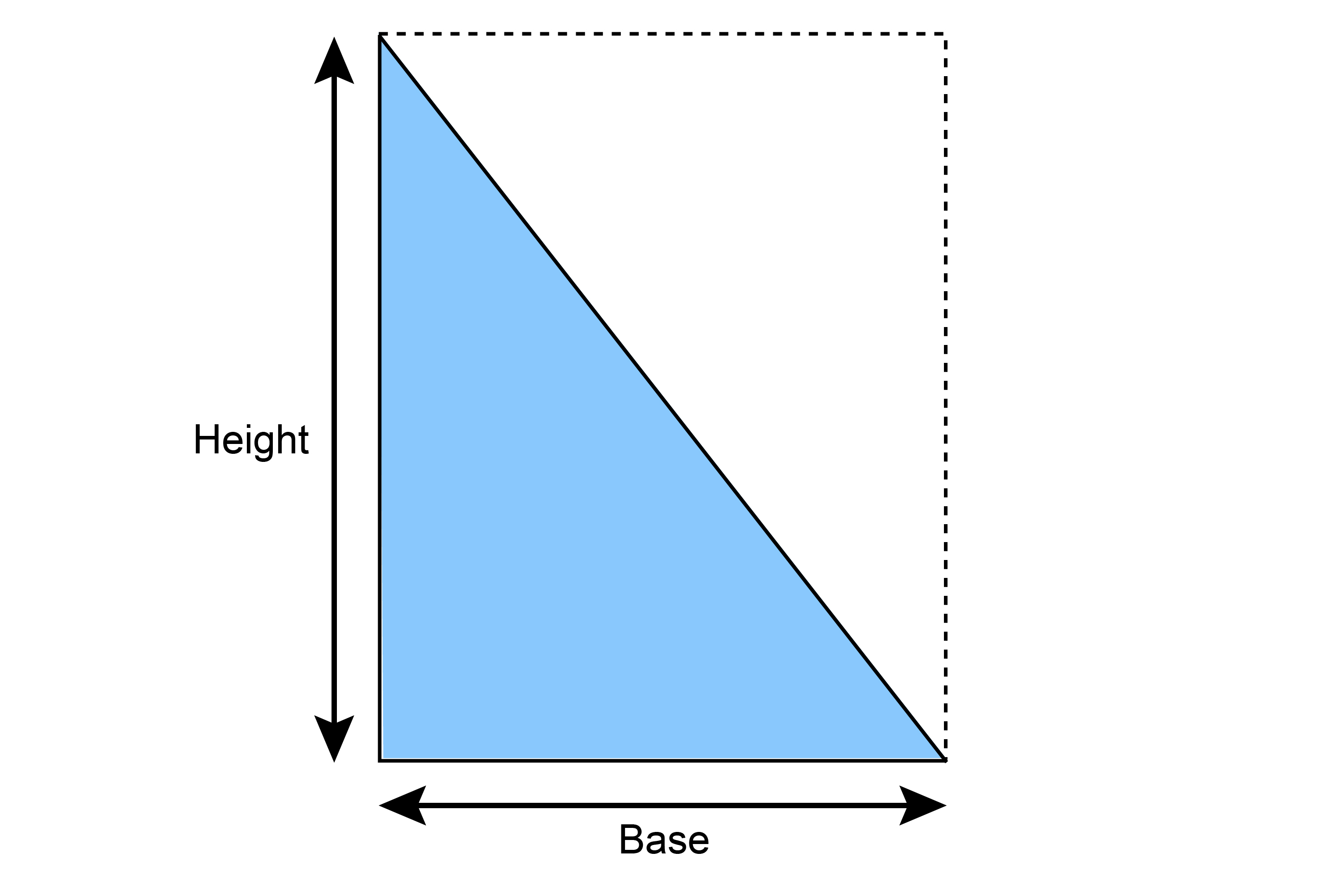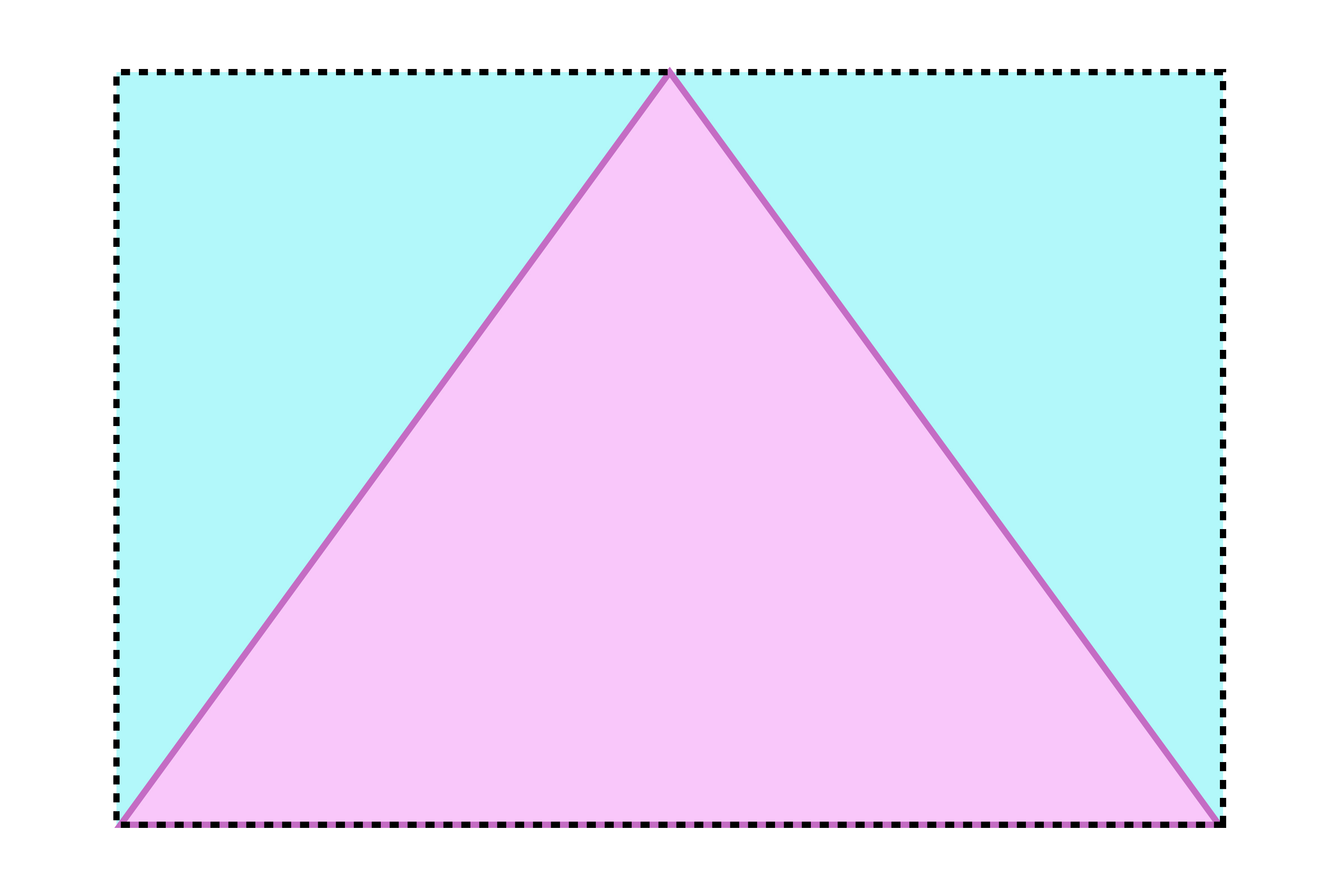# Area of a triangle

Area of a triangle - It's all about rectangles

Area of a triangle =1/2timesbasetimesheightThe area of a triangle is half of a rectangle.

So all you need to remember for the area of a triangle is the base times the height (as if it were a rectangle) then half it.

This works for any type of triangleTo remember the area of a triangle, imagine a rectangle around it.

In the diagram above, if you cut out the three triangles, the two outside triangles will fit exactly into the middle triangle.

Area of a triangle - Always imagine a rectangle around it